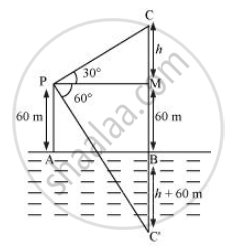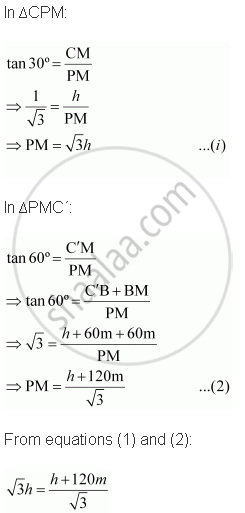# The Angle of Elevation of a Cloud from a Point 60 M Above the Surface of the Water of a Lake is 30° and the Angle of Depression of Its Shadow in Water of Lake is 60°. Find the Height of the Cloud from the Surface of Water - Mathematics

The angle of elevation of a cloud from a point 60 m above the surface of the water of a lake is 30° and the angle of depression of its shadow in water of lake is 60°. Find the height of the cloud from the surface of water

#### Solution

Let AB be the surface of the lake and P be the point of observation such that AP = 60 m. Let C be the position of the cloud and C be its reflection in the lake

Then CB = C'B

Draw PM⊥CB

Let CM = h

∴ CB = + 60 mFrom equations (1) and (2):

sqrt3h = (h + 120m)/sqrt3

⇒ 3h = h + 120 m

⇒ 2h = 120 m

⇒ h = 60 m

CB = h + 60m = 60m + 60m = 120m

Thus, the height of the cloud from the surface of the lake is 120 m

Concept: Heights and Distances
Is there an error in this question or solution?